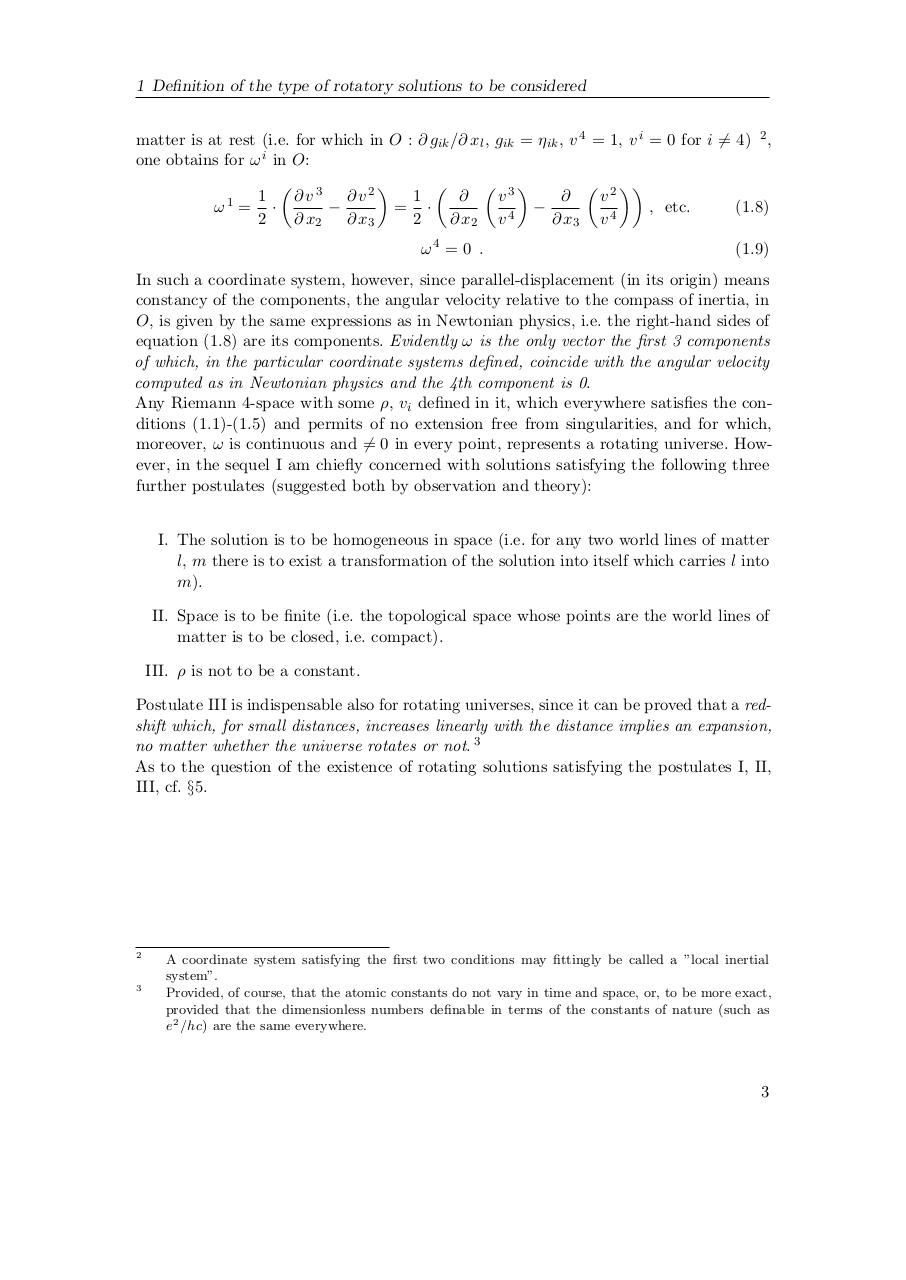# Kurt Gödel. Rotating Universes In General Relativity Theory.pdfPage 1 2 3 4 5 6 7 8 9 10 11

#### Text preview

1 Definition of the type of rotatory solutions to be considered
matter is at rest (i.e. for which in O : ∂ gik /∂ xl , gik = ηik , v 4 = 1, v i = 0 for i 6= 4) 2 ,
one obtains for ω i in O:

3
2
3

1
∂ v2
1
v

v
∂v

ω1 = ·

= ·

, etc.
(1.8)
2
∂ x2
∂ x3
2
∂ x2 v 4
∂ x3 v 4
ω4 = 0 .

(1.9)

In such a coordinate system, however, since parallel-displacement (in its origin) means
constancy of the components, the angular velocity relative to the compass of inertia, in
O, is given by the same expressions as in Newtonian physics, i.e. the right-hand sides of
equation (1.8) are its components. Evidently ω is the only vector the first 3 components
of which, in the particular coordinate systems defined, coincide with the angular velocity
computed as in Newtonian physics and the 4th component is 0.
Any Riemann 4-space with some ρ, vi defined in it, which everywhere satisfies the conditions (1.1)-(1.5) and permits of no extension free from singularities, and for which,
moreover, ω is continuous and 6= 0 in every point, represents a rotating universe. However, in the sequel I am chiefly concerned with solutions satisfying the following three
further postulates (suggested both by observation and theory):
I. The solution is to be homogeneous in space (i.e. for any two world lines of matter
l, m there is to exist a transformation of the solution into itself which carries l into
m).
II. Space is to be finite (i.e. the topological space whose points are the world lines of
matter is to be closed, i.e. compact).
III. ρ is not to be a constant.
Postulate III is indispensable also for rotating universes, since it can be proved that a redshift which, for small distances, increases linearly with the distance implies an expansion,
no matter whether the universe rotates or not. 3
As to the question of the existence of rotating solutions satisfying the postulates I, II,
III, cf. §5.

2

3

A coordinate system satisfying the first two conditions may fittingly be called a ”local inertial
system”.
Provided, of course, that the atomic constants do not vary in time and space, or, to be more exact,
provided that the dimensionless numbers definable in terms of the constants of nature (such as
e 2 /h c) are the same everywhere.

3